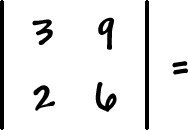A determinant (which uses vertical lines instead of brackets)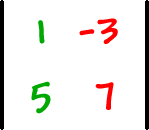will give us a number that goes along with the matrix.  We'll be able to use these numbers to solve systems!  Woo hoo!

OK, so here's how you get the determinant -- it's really easy: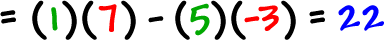Here's what I did:

I multiplied down this diagonal...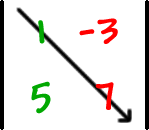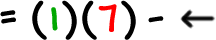Put a minus...

then multiplied up this diagonal...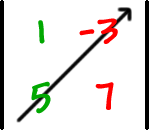Here's the general formula: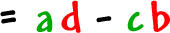TRY IT: